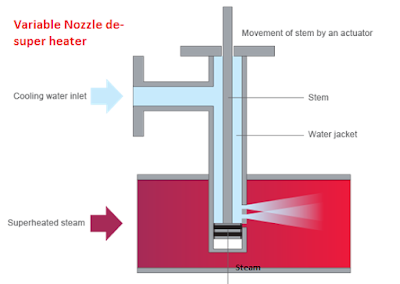### How do you calculate power plant efficiency???

Efficiency of the power plant is calculated based on heat input and out put.

Power plant efficiency = Heat out put X 100 / Heat out put

In power plant, generally heat input is fuel, make up water and condensate return water from process.

Heat out put is power generation.

The formula for efficiency calculation is different for different plants.

Efficiency of the power plant is given as

Efficiency = 860 X 100 / Heat rate

1-For thermal power plants efficiency is calculated as;

Efficiency = 860 X Power generation / Heat input

Efficiency = 860 X PG X 100 / (Fuel consumption X Fuel GCV)

2-For co generation plants heat rate is calculated as;

Efficiency = 860 X Power generation X 100 / (Fuel consumption X GCV + Make up water X Make up water enthalpy + Return condensate water X Enthalpy-Process steam flow X Enthalpy)

3-Cogen plant Efficiency is also calculated as;

Efficiency = 860 X Boiler efficiency X 100 / Turbine heat rate

Calculation:

1.Calculate the efficiency of power plant having heat rate 3451 Kcal/kwh

Efficiency of the power plant = 860 X 100 / Heat rate

Efficiency of the power plant = 860 X 100 / 3451

Efficiency of the power plant = 24.92%

Read power plant O&M reference books

2.A 75 MW Thermal power plant consumes 1100 MT coal per day, calculate the plant efficiency, consider the GCV of coal 5000 Kcal/kg

Efficiency of the plant = 860 X 24 X 75 X 100 / (1100 X 5000)

Efficiency of the plant = 28.15%

3. A 29 MW thermal power plant is having Turbine of heat rate 2798 kcal/kwh, which consumes steam at the rate of 4.2 MT/MW from a boiler having efficiency 69%.Calculate the efficiency of power plant

Efficiency = 860 X Boiler efficiency X 100 / Turbine heat rate

Efficiency = 860 X 0.69 X 100 / 2798

Efficiency = 21.2%

4. A 30 MW biomass Co generation plant consumes 1750 MT fuel per day and supplies 2880 TPH steam to process at pressure 2 kg/cm2 & 135 deg C, in turn receives 90% return condensate at 119 deg C temperature.The make up water for this plant is 610 m3 at temperature 27 deg C.Calculate the efficiency of the plant, consider GCV of biomass fuel 2250 kcal/kg.

Efficiency of Co-gen plant  = 860 X Power generation X 100 / (Fuel consumption X GCV + Make up water X Make up water enthalpy + Return condensate water X Enthalpy-Process steam flow X Enthalpy)

Enthalpy of process steam at 2 kg/cm2 and 135 deg C is 652 kcal/kg

Enthalpy of return condensate water is 121 kcal/kg

Enthalpy of make up water at 27 deg C is 27.2 kcal/kg

Efficiency of Co-gen plant  = 860 X 30 X 24 X 100 / (1750 X 2250 + 610 X 27.2 + 2880 X 80% X 121 X 2880 X 652)

Efficiency of Co-gen plant  = 860 X 30 X 24 X 100 / (1750 X 2250 + 610 X 27.2 + 2880 X 80% X 121 - 2880 X 652)

Efficiency of Co-gen plant = 26.29%

5. A thermal power plant operating at 125 MW operating  at pressure 110 kg/cm2 and temperature 545 deg C.A AFBC boiler of efficiency 84% consumes 379 TPH Feed water at temperature 200 degC to generate 375 TPH steam.Calculate the efficiency of the thermal power plant.

We have, Turbine heat rate = (Inlet steam flow X Enthalpy-Feed water flow X Enthalpy) / Power generation

Turbine heat rate = 375 X 821-379 X 202) / 125

Turbine heat rate = 1850.5 kcal/kwh

Turbine efficiency = 860 X 100 / 1850.5

Turbine efficiency = 46.5%

Power plant efficiency = Turbine efficiency X Boiler efficiency = 46.5 X 0.84 =39.06%

For more calculations read Powerplant and calculations